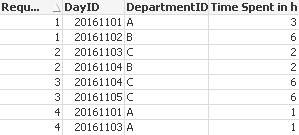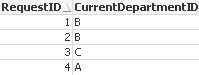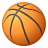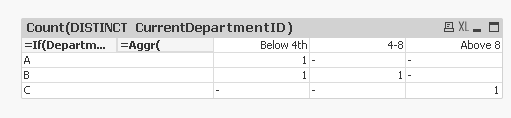# QlikView App Dev

Discussion Board for collaboration related to QlikView App Development.

Announcements
Skip the ticket, Chat with Qlik Support instead for instant assistance.
cancel
Showing results for
Did you mean:Not applicable

## SetAnalysis issue - how to compare two dimensions

Dear Community,

I'm new person here still learning new thing, and I'm struggling with SetAnalysis function.

I have set of data - fact table storing how much time each request spend in departmentAnd dimension, saying to which department this request is currently signed.What i need to find is number of request which are in current department for defined period of time ( below 4 hours, 4-8 hours, above 8 hours).

So i would like to get following results:

DeptBelow 4h 4-8Above 8
A1
B11
C1

I tried to write sum function, calculating time spent only for current department, but such syntax doesn't return me correct values:

Sum({\$<DayID-=P({1<DepartmentID={\$(=CurrentDepartmentID)}>}DayID)>}[Time Spent in h])

I'm attaching file with simple data.

I'll be very gratefull for your help.

Regards,
Greg

1 Solution

Accepted SolutionsMVP

In that case, may be you want to do a distinct count of RequestID

Count(DISTINCT RequestID)10 RepliesPartner - Master II

DepartmentID=CurrentDepartmentID should do although it will appear red as if the expression is wrongNot applicable
Author

I marked it red - just to point, that this is part cause problems here.
And it's not working, it returns normal sum.Anonymous
Not applicable
Author

Hi,

Dimension 1:- =if(DepartmentID=CurrentDepartmentID,CurrentDepartmentID)

Dimension 2:- =if([Time Spent in h]<4,'Below 4th',if([Time Spent in h]<8,'4-8','Above 8'))

Expression:- Count(DISTINCT DayID)

Thanks,Not applicable
Author

Hi,

Unfortunately it doesn't show correct values.

It check time spend for each day separately per DayID, and i need to check total time spent in last Department.

eg. RequestID=3 been 2 days in department C, together it is 6+6=12 hours and should be counted as 'Above 8 hours'Anonymous
Not applicable
Author

Hi Mroz,

For following result, please find attached QVW and dimension and expressions.

 Dept Time1 Above 8 4-8 Below 4th A - - 1 B 1 1 - C 1 - -

Dimension 1:- =if(DepartmentID= CurrentDepartmentID, CurrentDepartmentID)

Dimension 2:- =aggr( if(sum([Time Spent in h])<4,'Below 4th',if(sum([Time Spent in h])<8,'4-8',

if(sum([Time Spent in h])>8,'Above 8'))),RequestID)

Expression:- Count(DISTINCT CurrentDepartmentID)

Thanks & Regards,

Pooja SinghAnonymous
Not applicable
Author

Hi Mroz,

For following result, please find attached QVW and dimension and expressions.

 Dept Time1 Above 8 4-8 Below 4th A - - 1 B 1 1 - C 1 - -

Dimension 1:- =if(DepartmentID= CurrentDepartmentID, CurrentDepartmentID)

Dimension 2:- =aggr( if(sum([Time Spent in h])<4,'Below 4th',if(sum([Time Spent in h])<8,'4-8',

if(sum([Time Spent in h])>8,'Above 8'))),RequestID)

Expression:- Count(DISTINCT CurrentDepartmentID)

Thanks & Regards,

Pooja SinghMVP

May be you need this:

Dimension

=If(DepartmentID = CurrentDepartmentID, CurrentDepartmentID)

=Aggr(

If(Sum([Time Spent in h]) < 4, Dual('Below 4th', 1),

If(Sum([Time Spent in h]) < 8, Dual('4-8', 2),

If(Sum([Time Spent in h]) > 8, Dual('Above 8', 3)))), RequestID, DepartmentID)

Expression

Count(DISTINCT CurrentDepartmentID)Not applicable
Author

Hi Sunny,

It's almost perfect.

But it don't count request.

When i add another request do Department C (with id=5) - i still see 1 request below 4th in dept. C (insted of two), see example:MVP

In that case, may be you want to do a distinct count of RequestID

Count(DISTINCT RequestID)Community Browser